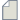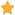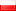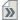###Author Topic: Raman Spectra  (Read 338 times)

0 Members and 1 Guest are viewing this topic.

####wjjan25

• New ATK user
•• Posts: 2
• Country:• Reputation: 0##### Raman Spectra
« on: July 12, 2021, 16:14 »
Hello,

I am a summer student working on ab initio computation of Raman spectra -  I am new to QuantumATK.

I went through the documentation section and the theory outlined here:
https://docs.quantumatk.com/manual/Types/RamanSpectrum/RamanSpectrum.html

However, besides the theory itself, I have been wondering how the evaluation of the Raman tensor and the susceptibility derivatives has been implemented.

In particular, what interests me the most is how the susceptibility tensor is computed using the Kubo-Greenwood formula, i.e. how the momentum matrix elements are calculated (and how they are called by the rest of the code) and what broadening (gamma) is used, both of which were mentioned in the documentation: https://docs.quantumatk.com/manual/Types/OpticalSpectrum/OpticalSpectrum.html

Would it be possible at all to ask for some indication? I would be extremely happy if by any chance it would be possible to analyse some bit of the code related to this part of the implementation.

Thanks a lot, any help is very much appreciated!

####wjjan25

• New ATK user
•• Posts: 2
• Country:• Reputation: 0##### Re: Raman Spectra
« Reply #1 on: July 16, 2021, 16:48 »
Hello,

Just to follow up my query - beyond QuantumATK I have recently tried using GPAW code integrated with ASE (https://wiki.fysik.dtu.dk/gpaw/tutorials/elphraman/elphraman.html?fbclid=IwAR1a-qGnbwyGFcEmi0oegE5OfPold1ndlpzzHcy2PXLx2vrbj3ixfIuyELU) where from the documentation it follows how the momentum matrix elements for Raman spectra are computed and called.

Even though the implementations of Raman spectra in both codes are different (as far as I know in QuantumATK momentum matrix elements are used to find susceptibility tensor from the Kubo-Greenwood formula, while GPAW uses these to compute Raman tensor directly as follows from third-order Fermi's golden rule) - I am still wondering how to call the momentum matrix elements in QuantumATK and how these are implemented.

I have found out how to call the susceptibility derivatives when computing Raman spectra, but I am totally clueless about how susceptibility tensor calls momentum matrix elements when it is evaluated by OpticalSpectrum object: https://docs.quantumatk.com/manual/Types/OpticalSpectrum/OpticalSpectrum.html

Would it be possible to ask for some hint?

Thanks for any potential help!

####Tue Gunst

• Regular ATK user
•• Posts: 36
• Country:• Reputation: 2##### Re: Raman Spectra
« Reply #2 on: August 3, 2021, 10:59 »
The momentum matrix elements is available as a low level entity in QuantumATK and can be accesses in the following way:
"
p_matrix = calculateMomentumMatrixElements(
configuration,
kpoint=None,
number_of_states=None):
"
The function calculates the momentum matrix elements for a bulk configuration at a specified k-point (fractional).
The x-component of the momentum matrix elements is defined as
p_nm(k) = <mk | -i * hbar * d/dx | nk>,
where |nk> is a Bloch state in band 'n' at the k-point 'k', and likewise for the y- and z-components.
For Unpolarized, Noncolliner and Spin-orbit calculations, each matrix has a shape (3, *N*, *N*), where *N* is the number of bands included.
For Polarized calculations, the shape is (2, 3, *N*, *N*) with the first index corresponding to Spin.Up and Spin.Down.